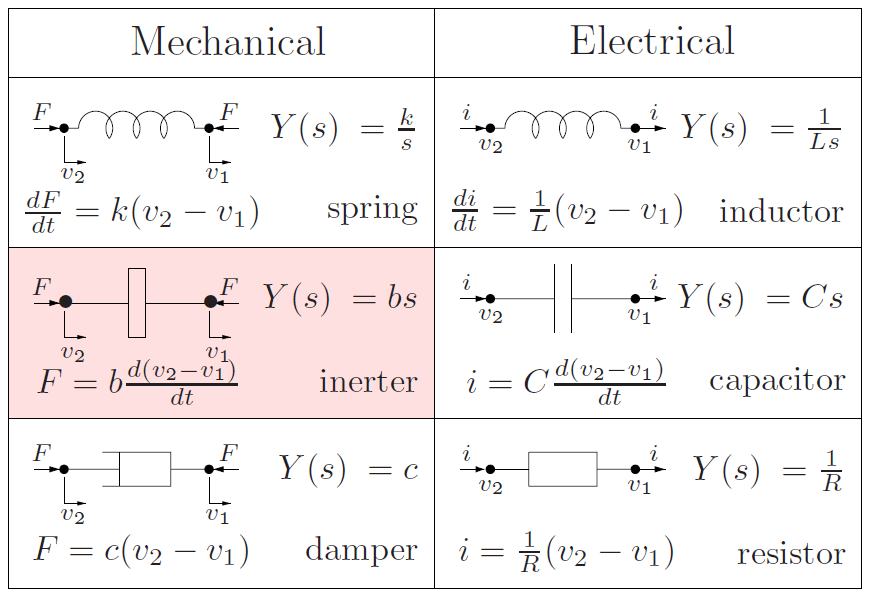# Self-Inductance

• Self-inductance (L) is the ability of a circuit to oppose the magnetic flux that is produced by the circuit itself

• Running a changing current through a circuit creates a changing magnetic field, which creates an induced emf that fights the change

• Units are henrys (H)

•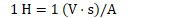• Self-inductance is purely a function of the circuit's geometry

# Calculating Self Inductance

•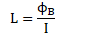• Ratio of magnetic flux to current flow

•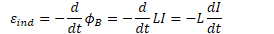• For inductor:

•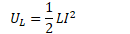• For capacitor:

•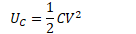# Example 1: Self Inductance of s Solenoid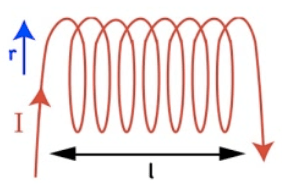• Calculate the self-inductance of a solenoid of radius r and length L with N windings

•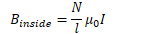•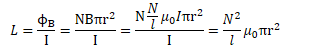# Example 2: Calculating Self Inductance

• Calculate the self-inductance of a solenoid with 3400 turns of wire if the solenoid is 9 cm long and has a diameter of 11 cm.

•# Inductor, Capacitor, and Resistor